Courses

# Vapour Compression Systems, Refrigerants - MCQ Test 2

## 30 Questions MCQ Test GATE Mechanical (ME) 2022 Mock Test Series | Vapour Compression Systems, Refrigerants - MCQ Test 2

Description
This mock test of Vapour Compression Systems, Refrigerants - MCQ Test 2 for Mechanical Engineering helps you for every Mechanical Engineering entrance exam. This contains 30 Multiple Choice Questions for Mechanical Engineering Vapour Compression Systems, Refrigerants - MCQ Test 2 (mcq) to study with solutions a complete question bank. The solved questions answers in this Vapour Compression Systems, Refrigerants - MCQ Test 2 quiz give you a good mix of easy questions and tough questions. Mechanical Engineering students definitely take this Vapour Compression Systems, Refrigerants - MCQ Test 2 exercise for a better result in the exam. You can find other Vapour Compression Systems, Refrigerants - MCQ Test 2 extra questions, long questions & short questions for Mechanical Engineering on EduRev as well by searching above.
QUESTION: 1

### The values of enthalpy at the beginning of compression, at the end of compression and at the end of condensation are 185 kJ/kg, 210 kJ/kg and 85 kJ/kg respectively. What is the value of the COP of the vapour compression refrigeration system?

Solution: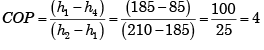QUESTION: 2

Solution:
QUESTION: 3

### onsider the following statements in respect of absorption refrigeration and vapour compression refrigeration systems: 1. The former runs on low grade energy. 2. The pumping work in the former is negligible since specific volume of strong liquid solution is small. 3. The latter uses an absorber while former uses a generator. 4. The liquid pump alone replaces compressor of the latter.   Which of these statements are correct?

Solution:
QUESTION: 4

For a heat pump working on vapour compression cycle, enthalpy values of the working fluid at the end of heat addition process, at the end of compression process, at the end of heat rejection process, and at the end of isenthalpic expansion process are 195 kJ/kg, 210 kJ/kg, and 90 kJ/kg respectively. The mass flow rate is 0.5 kg/s. Then the heating capacity of heat pump is, nearly

Solution:
QUESTION: 5

In a vapour compression plant, if certain temperature differences are to be maintained in the evaporator and condenser in order to obtain the necessary heat transfer, then the evaporator saturation temperature must be:

Solution:
QUESTION: 6

In milk chilling plants, the usual secondary refrigerant is:

Solution:
QUESTION: 7

A single-stage vapour compression refrigeration system cannot be used to produce ultralow temperatures because

Solution:
QUESTION: 8

The vapour compression refrigeration cycle is an inherently irreversible cycle, because

Solution:
QUESTION: 9

Consider the following statements pertaining to a vapour compression type refrigerator:

1.The condenser rejects heat to the surroundings from the refrigerant.

2.The evaporator absorbs heat from the surroundings to be cooled.

3.Both the condenser and evaporator are heat exchangers with refrigerant as a common medium.

4.The amount of heat exchanged in condenser and evaporator are equal under steady conditions.

Which of the above statements are correct?

Solution:
QUESTION: 10

The correct sequence of vapour compression (VC), vapour absorption (VA) and steam ejector (SE) refrigeration cycles in increasing order of the COP is:

Solution:

The correct sequence of VC, VA and SE in increasing order of COP is VA, SE and VC, the Value being of the order of 0.3 to 0.4 0.5 to 0.8 and 4 to 5 respectively.

QUESTION: 11

The leakage in a Freon-based refrigeration system can be detected by using a/an

Solution:
QUESTION: 12

Consider the following statements:

High condenser pressure in a refrigeration system can occur because

1. The water flow rate is lower than the desired value.

2. Non-condensable gases are present in the system

3. Of accumulation of lubricating oil in condenser

4. Of low charge of refrigerant in the system.

Of these statements:

Solution:
QUESTION: 13

In system A vapour are superheated by 10°C in the evaporator while in system B vapour are superheated by 10°C in a liquid vapour regenerative heat exchanger, other conditions being the same. Then

Solution:

h1′ −h1= h3 − h3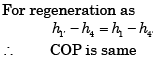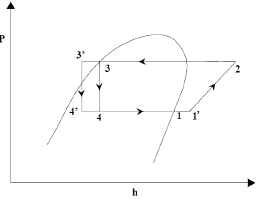QUESTION: 14

Selection of a refrigerant for a vapour – compression system depends on which among the following?

Solution:
QUESTION: 15

Oil separator is NOT required in refrigeration system if:

Solution:
QUESTION: 16

Consider the following statements:

Moisture should be removed from refrigerants to avoid

1. Compressor seal failure

2. Freezing at the expansion valve

3. Restriction to refrigerant flow

4. Corrosion of steel parts

Of these statements:

Solution:

All the statements about effect of moisture on refrigerant are correct.

QUESTION: 17

ssertion (A): R-22 is used as a refrigerant in all refrigerators.

Reason (R):  R-22 is non-toxic and non-inflammable.

Solution:
QUESTION: 18

Match List-I with List-II and select the correct answer using the codes given below the lists: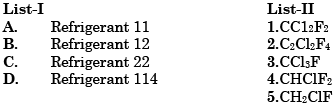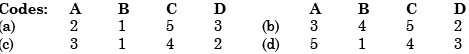Solution:

R (C − 1) ( H+1) F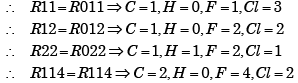QUESTION: 19

Assertion (A): Freon-12 is odourless and its leakage cannot be easily detected. However, it is preferred in comfort air-conditioning.

Reason (R): It is almost impossible for Freon-12 leakage to attain a fatal concentration.

Solution:
QUESTION: 20

Match List-I with List-II and select the correct answer using the codes given below the lists: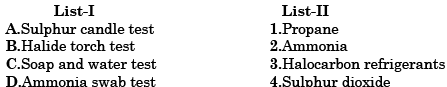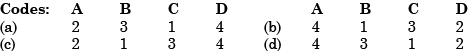Solution:
QUESTION: 21

The color of the flame of halide torch, in a case of leakage of Freon refrigerant, will change to:

Solution:
QUESTION: 22

What is an azeotrope?

Solution:

Azeotrope is a mixture of refrigerants without phase separation.

QUESTION: 23

The leaks in a refrigeration system freon are detected by:

Solution:

Several methods are available for the detection of leaks. The most common is the soap-bubble method. The other is the halide torch method used with fluorocarbons.

QUESTION: 24

Match List-I (Chemical formula of refrigerant) with List-II (Numerical Designation) and select the correct answer using the codes given below the lists: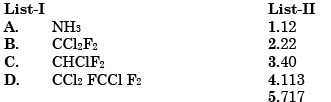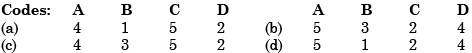Solution:

R(C – 1)(H + 1)F and Cl by balance and for inorganic refrigerant R(700 + Molecular weight).

QUESTION: 25

Match List-I (Refrigerant) with List-II (Designation) and select the correct answer using the codes given below the lists: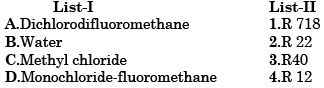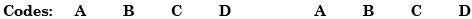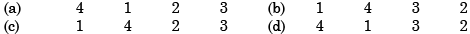Solution:
QUESTION: 26

Theoretical vapour compression refrigeration cycle is represented on a T-s diagram as

Solution:
QUESTION: 27

Match List-I (T-s diagram) with List-II (P-h diagrams) of vapour compression refrigeration cycles and select the correct answer using the codes given below the lists: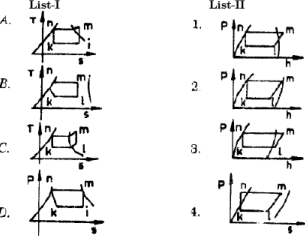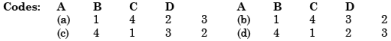Solution:
QUESTION: 28

Replacing a water-cooled condenser with an air-cooled one in a vapour compression refrigeration system with constant evaporator pressure results in

Solution:

Heat transfer co-efficient of gas very small compared to water hwater >> hair. So for same heat transfer temperature difference will be high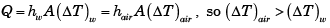QUESTION: 29

The power required for the compressor in kw is

Solution:

Correct Answer :- c

Explanation : Since power is required for compressor in refrigeration is in compression cycle (1-2)

Hence, Power required = m (h2 -h1) = mo (h2 -hf)

Since for isentropic compression process.

s1 = s2 from figure. = 0.95

For entropy s = 0.95 the enthalpy h = 276.45 kJ /kg

h = h2 = 276.45 (From table)

Hence Power = 0.2(276.45 -237)

= 7.9 kW

QUESTION: 30

A good refrigerant should have

Solution: